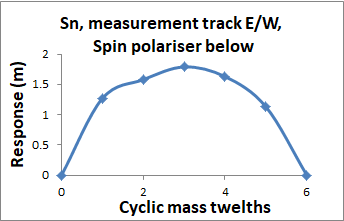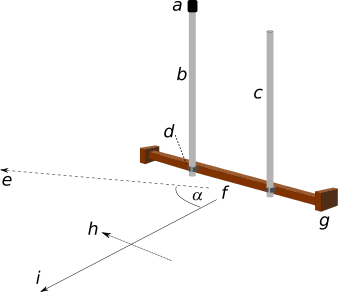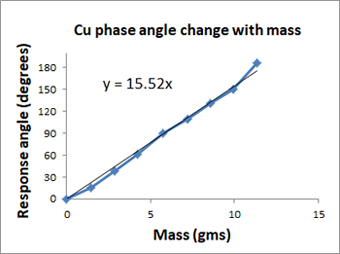# spinTorsion

## 1. Summary

An isolated mass of a chemical element when irradiated by spin torsion radiation from the Sun, causes a detectable fringe with the fringe distance being some function of both the element and the mass. When the mass is increased the distance from the mass to the fringe that it creates increases to some maximum value, decreases to zero and then repeats the expansion and contraction in a cyclical manner. Every element has a unique value of mass that causes one cycle of contraction and expansion. This mass has been called the cyclic mass of an element.

Not all the chemical elements behave in quite the same way. Early work found that elements fall into one of two categories depending on the way in which the detector rods are held and respond to an isolated mass. These categories have been called the red blue group categories.

Experiments reported here use a vertical tube interferometer and shed new light onto the nature of cyclic mass and the categorisation of elements into red and blue groups.

A vertical tube interferometer allows the radiation wavefront direction to be determined using detector rods. It was found that as mass was added to one of the interferometer tubes the radiation direction changed. When a cyclic mass was added the direction changed through 180 °. It was found that elements belonging to the red group changed the direction one way and elements from the blue group the other way.

The opposite ways in which red group and blue group elements change the radiation direction provides a new understanding of neutral materials. These are materials that are created by combining material from elements in the red and blue groups in proportion to their cyclic masses so that their influence wavefront direction cancels.

## 2. Introduction

It has previously been observed that a small mass (an isolated mass) of an element when placed on a plain surface such as a floor at a reasonable distance (say 1m) from surrounding objects generated fringes that can be found using detector rods. When using such rods there are two ways to hold them. The work reported on this web site uses what is known as a "Thumbs up" hold in which the thumb sticks up behind the bend in the rod. This is the hold shown in the diagram on the resources page. There is an alternative hold in which the thumb is pointed forward and clasped around the first finger. This is known as the "thumbs round" hold.

When using rods to investigate the fringes created by an isolated mass it has previously been found that the fringes created by small masses of some elements could only be detected using the thumbs up hold, other elements could only be detected using thumbs down. In consequence elements were then categorised as belonging to one of two groups. These groups are currently designated the red and blue group.

A.N.Coll (personal communication) found that when a red type material was combined in appropriate proportions with a blue type material the result was a material that did not create any detectable fringes regardless of its mass. He called this combination a neutral material.

A.N.Coll (personal communication) also found that when the mass of an element under investigation was incremented and the fringe distance measured at each increment, the fringe distance changed from near zero for a very small mass, increased to some maximum value and then returned to zero. The mass that was required to complete this cycle was called the cyclic mass. He found that the cyclic mass for a given element was unique.

At around the same time that A.N.Coll was working with cyclic mass, the author found that the fringe created by the interferometer varied in distance in a cyclic manner when mass was added to one of the tubes. This behaviour together with the use of a vertical interferometer has led to a new understanding of cyclic mass and neutral materials.

## 3. Isolated mass response

This experiment is a partial replication of experiments carried out by A .N. Coll in order to determine the cyclic mass of elements. He defined the cyclic mass as the mass needed to go from zero fringe distance response and back to zero.

In the earlier work the results indicated that the cyclic mass function was a triangular or sawtooth function. However the present author has found that earlier measurements were significantly influenced by polarisation effects from the mass of the planet below the experimental area. These effects could be minimised by covering the floor of the experimental area with a sheet of polyethylene polariser material with its stretch direction aligned north/south.

An experiment was carried out to determine the cyclic mass of tin with the floor cover in place. Rather than use random masses of tin, masses that were a proportion of a cyclic mass were used, these having been calculated by using cyclic mass values provided by A. N. Coll.  A mass of tin was placed on the floor on top of the polarising sheet. Using detector rods the experimenter walked away from it until the rods crossed. The distance was noted, the mass of tin was increased and the measurement repeated.

######Figure 1. A half cyclic mass function

Figure 1 shows the result. The response curve goes from zero, up to a maximum and down to zero for what was previously believed to be a cyclic mass of tin. However because of results from the vertical tube interferometer detailed below, what was previously believed to be a cyclic mass appears to only be a half cyclic mass. As a result the horizontal axis of fig. 1 has been changed to reflect this.

## 4. Adding mass to an interferometer tube.

######Figure 2.  Vertical interferometer with added mass

Fig. 2 shows the arrangement of a vertical interferometer used to study the effect of mass.

The interferometer comprised a wooden support g 2cm x 1.2cm x 78cm wide. The support had wooden ends 14cm wide x 8cm x 2cm. These dimensions were not believed to be critical.

Two Aluminium tubes b and c of diameter 1.5cm and height 52.75cm were mounted in a vertical position with a spacing of 10.55 cm using nylon pipe clips d

The line f-i shows the radiation direction when there was no added mass at a. The line f-e shows the new radiation direction when mass was added at a.

These lines could be found by the crossing of detector rods at right angles to the radiation direction as shown at h for direction f-i.

When mass was added at a on the top of tube b the angle of radiation emission α could be changed from its no-added-mass direction f-i to some other direction shown as f-e. Elements such as copper which is in the red group caused the angle to increase in a clockwise direction and elements such as tin which are in the blue group caused the angle to increase in a counter clockwise direction. Note that if the mass was added to tube c the reverse was true. For any given element, angle α changed through 180° when a cyclic mass was added at a.

######Figure 3.  Angle change with added mass

Fig 3. shows the original curve and a straight line fitted to the data points. There appears to be a linear relationship between mass and the angle through which the radiation direction changes. The graph shows that for the copper wire used in the experiment the angle changed through 180° for a mass of 11.6gms of copper. In the past this has been taken as the cyclic mass. However a better definition of cyclic mass is the mass required to cause the radiation direction to go through 360° rather than the 180° in fig. 3. Thus the true cyclic mass of the copper wire should be 23.2gms (2 x 11.6gms.)

## 5. Neutral materials

In fig.2 when a mass is added at position a at the top of tube b the mass influences the radiation direction of the interferometer. If a mass is added at position a and has no effect on the direction then the material is said to be neutral.

When a 1/3 cyclic mass of the element tin - a blue group element - was placed at position a it was found that the radiation direction changed in a counter clockwise direction by 1/3 of 360° from its direction with no mass at a. For a 1/3 cyclic mass of copper- - a red group element - the radiation changed in a clockwise direction by 1/3 of 360°

When the mass of tin and the mass of copper were placed together at position a it was found that the radiation direction reverted to its original no-mass-added condition in direction f - i in fig.1. The combination of 1/3 cyclic mass of tin in combination with 1/3 cyclic mass of copper is thus a neutral combination. The experimental results strongly suggested that any element in the red group combined with any element in the blue group in proportion to their cyclic masses would be neutral.

A limited range of pure elements in a limited range of weights were supplied by A. N. Coll.   Various combinations of pairs of these elements were tested to check if red and blue type elements combined in proportion to their cyclic masses were neutral. The test used to determine this was to place the combination at position a on top of tube b and check the radiation direction. If the radiation direction remained in direction f - i then the combination was considered to be neutral.

In table 1 each row in the table details a particular combination. All of the combinations in the table were found not to change the radiation direction by any measurable angle and were thus considered to be neutral. The cyclic mass values in the table were provided by A. N. Coll (personal communication) and have been retained rather than being doubled to their correct values.

Red Group Cyclic Mass (gm) % CM Blue Group Cyclic Mass (gm) % CM
Sulphur 7.99gm 50.0 Duralumin 12.18 49.8
Sulphur 7.99gm 25.4 Zinc 17.71 25.0
Lead 48.86 66.1 Duralumin 12.18 65.5
Lead 48.86 32.9 Zinc 17.71 33.4
Lead 48.86 16.7 Tin 26.33 16.6
Lead 48.86 33.2 Tin 26.33 33.6
Lead 48.86 66.1 Tin 26.33 67.3
Carbon 2.23 66.5 Duralumin 12.18 65.6
Carbon 2.23 33.3 Zinc 17.71 33.5
Copper 12.98 22.6 Zinc 17.71 22.6
Carbon 2.23 16.5 Tin 26.33 16.6
Carbon 2.23 32.3 Tin 26.33 33.6
Carbon 2.23 66.5 Tin 26.33 67.3
Copper 12.98 53.4 Duralumin 12.18 50
Copper 12.98 22.6 Zinc 17.71 22.6
Copper 12.98 33.7 Tin 26.33 33.6
Copper 12.98 67.1 Tin 26.33 67.3

Table 1 Neutral Mixtures

When mass was placed on top of one of the tubes of a vertical tube interferometer the angle of radiation emission from the interferometer swept around in a horizontal circle as the mass was increased. For the original definition of cyclic mass the angle swept through 180°. The new definition of cyclic mass is thus the mass that is required to sweep the angle through 360°

If the same interferometer is rearranged so that the tubes are horizontal, then as mass is added to one of the tubes the angle of radiation emission will sweep through a circle in the vertical plane. This leads to the view that the fringe distance for a horizontal tube interferometer is in some sense a projection on the planet's surface of the horizontal component of the vector describing the radiation direction for a particular mass.

For an isolated mass of an element the plot of fringe distance against cyclic mass takes the form of a half sine wave (fig. 1). This can also be thought of as the horizontal component of the vector describing the radiation direction for a particular mass except that rather than the radiation being in a circle in the vertical plane the radiation direction is in a sphere around the isolated mass because it radiates in all directions.

The angle of emission α of spin torsion from a vertical tube interferometer can be adjusted in more than one way.  The angle can be changed by adjusting the mass placed on top of one of the tubes as in this experiment. Another way, described in the frequency experiment is by adjusting the value of a resistor in a resistor inductor circuit that is connected in series with one of the interferometer tubes.

Neutral materials are used to eliminate the effect of structural elements and one material that has been used extensively is Brass. Brass is an alloy of Copper and Zinc. Although the proportions can be variable depending on the application that it is intended for, it has been found that the ratio of Copper to Zinc gives a reasonable approximation to a neutral material and that in general it does not seriously react in experimental setups. It has been used in tube and strip form for supporting interferometer elements and in wire form for connecting circuits.

## 6. Acknowledgements

The author wishes to acknowledge other investigators (who wish to remain anonymous) work on the discovery of cyclic mass and neutral materials and the provision of cyclic mass samples of various elements.